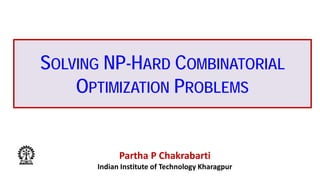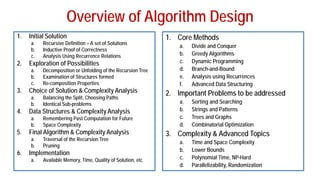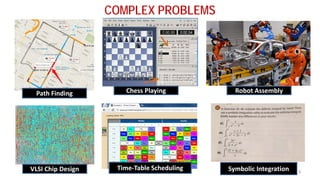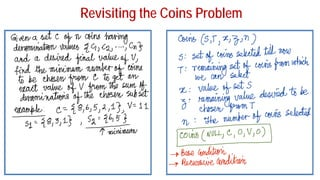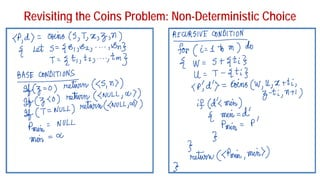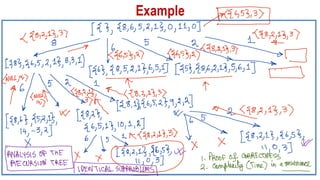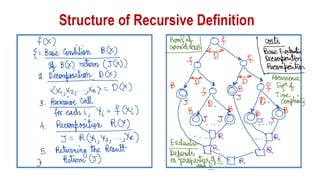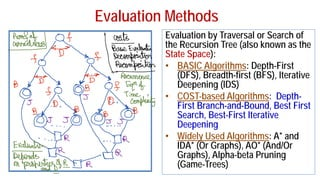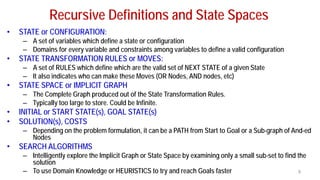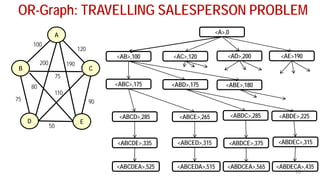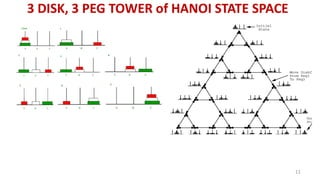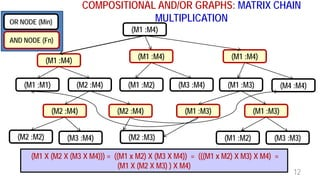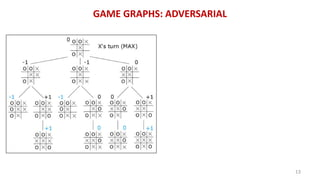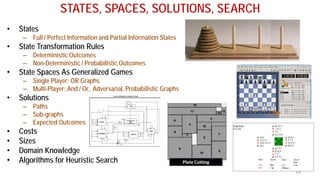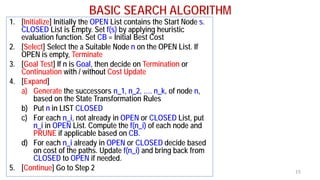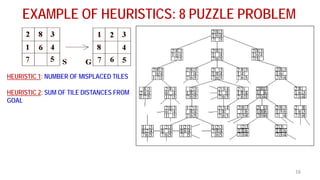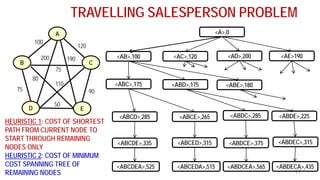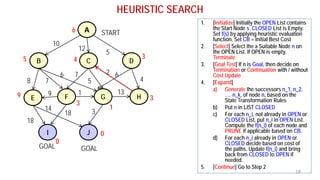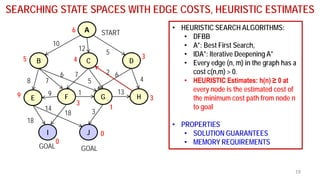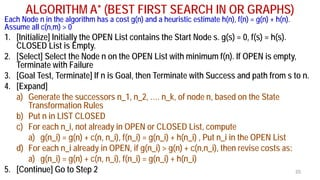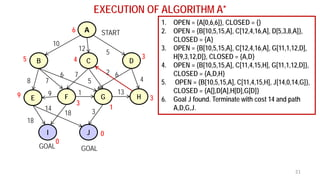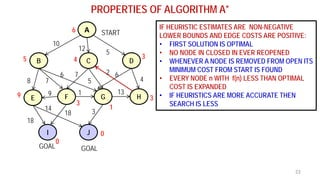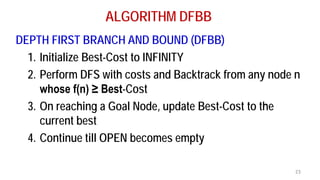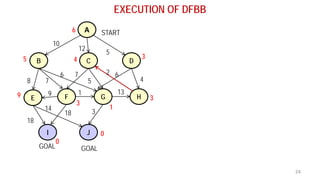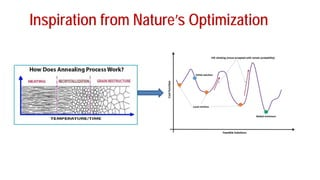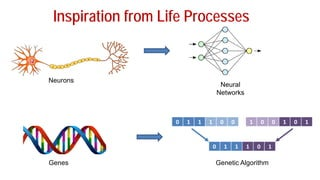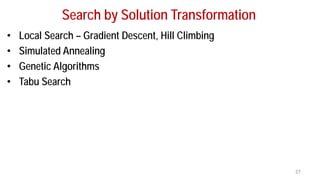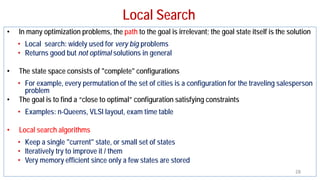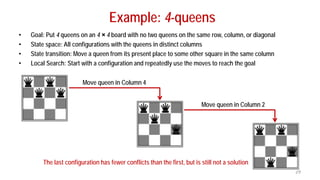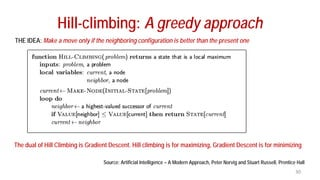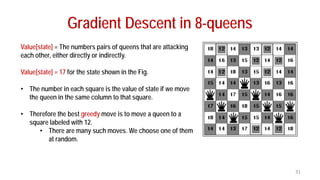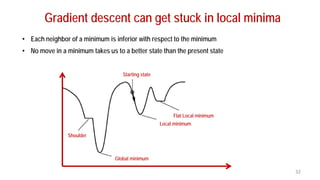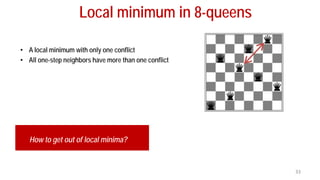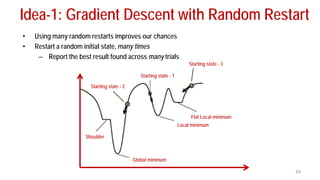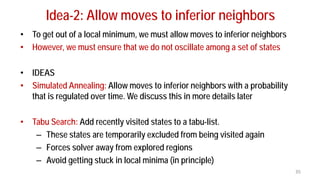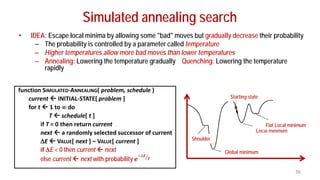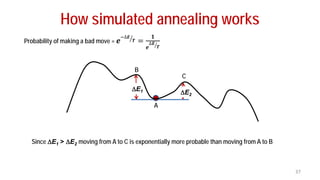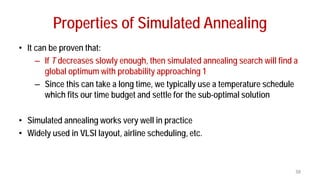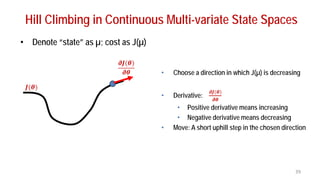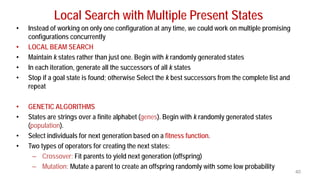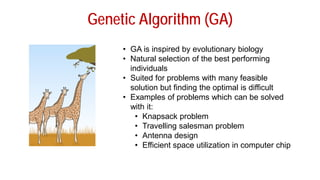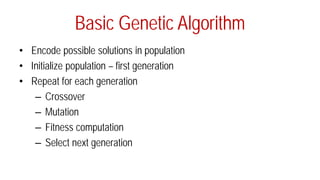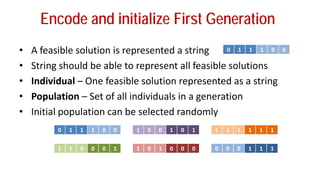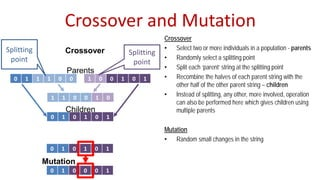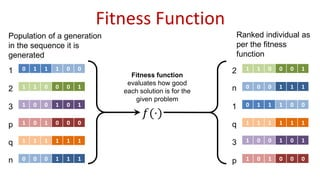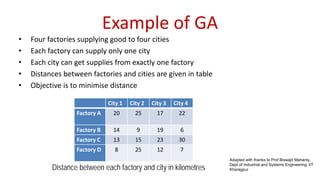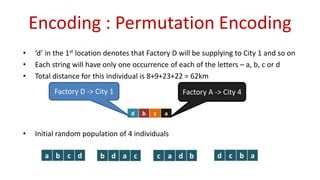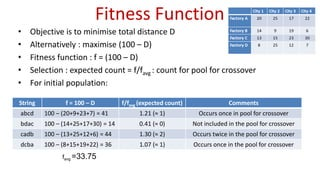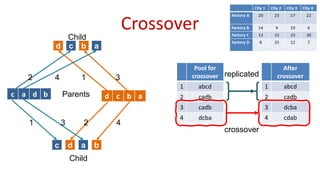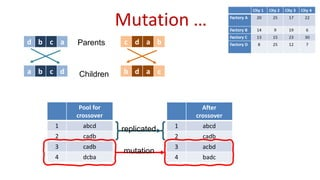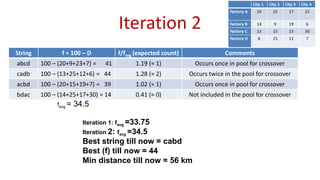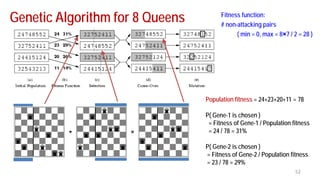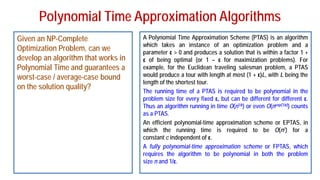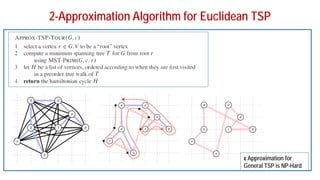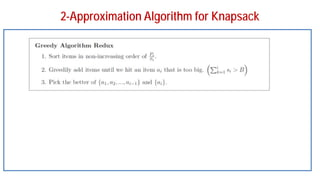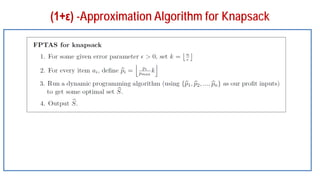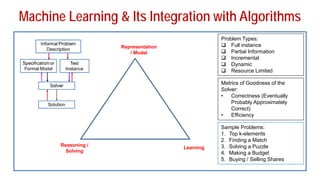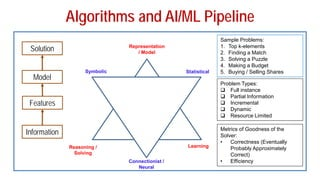1 of 59

### FADML 10 PPC Solving NP-Hard Problems.pdf

1. SOLVING NP-HARD COMBINATORIAL OPTIMIZATION PROBLEMS Partha P Chakrabarti Indian Institute of Technology Kharagpur
2. Overview of Algorithm Design 1. Initial Solution a. Recursive Definition – A set of Solutions b. Inductive Proof of Correctness c. Analysis Using Recurrence Relations 2. Exploration of Possibilities a. Decomposition or Unfolding of the Recursion Tree b. Examination of Structures formed c. Re-composition Properties 3. Choice of Solution & Complexity Analysis a. Balancing the Split, Choosing Paths b. Identical Sub-problems 4. Data Structures & Complexity Analysis a. Remembering Past Computation for Future b. Space Complexity 5. Final Algorithm & Complexity Analysis a. Traversal of the Recursion Tree b. Pruning 6. Implementation a. Available Memory, Time, Quality of Solution, etc 1. Core Methods a. Divide and Conquer b. Greedy Algorithms c. Dynamic Programming d. Branch-and-Bound e. Analysis using Recurrences f. Advanced Data Structuring 2. Important Problems to be addressed a. Sorting and Searching b. Strings and Patterns c. Trees and Graphs d. Combinatorial Optimization 3. Complexity & Advanced Topics a. Time and Space Complexity b. Lower Bounds c. Polynomial Time, NP-Hard d. Parallelizability, Randomization
3. COMPLEX PROBLEMS 3 Path Finding Chess Playing Robot Assembly VLSI Chip Design Time-Table Scheduling Symbolic Integration
4. Revisiting the Coins Problem
5. Revisiting the Coins Problem: Non-Deterministic Choice
6. Evaluation Methods Evaluation by Traversal or Search of the Recursion Tree (also known as the State Space): • BASIC Algorithms: Depth-First (DFS), Breadth-first (BFS), Iterative Deepening (IDS) • COST-based Algorithms: Depth- First Branch-and-Bound, Best First Search, Best-First Iterative Deepening • Widely Used Algorithms: A* and IDA* (Or Graphs), AO* (And/Or Graphs), Alpha-beta Pruning (Game-Trees)
7. Recursive Definitions and State Spaces • STATE or CONFIGURATION: – A set of variables which define a state or configuration – Domains for every variable and constraints among variables to define a valid configuration • STATE TRANSFORMATION RULES or MOVES: – A set of RULES which define which are the valid set of NEXT STATE of a given State – It also indicates who can make these Moves (OR Nodes, AND nodes, etc) • STATE SPACE or IMPLICIT GRAPH – The Complete Graph produced out of the State Transformation Rules. – Typically too large to store. Could be Infinite. • INITIAL or START STATE(s), GOAL STATE(s) • SOLUTION(s), COSTS – Depending on the problem formulation, it can be a PATH from Start to Goal or a Sub-graph of And-ed Nodes • SEARCH ALGORITHMS – Intelligently explore the Implicit Graph or State Space by examining only a small sub-set to find the solution – To use Domain Knowledge or HEURISTICS to try and reach Goals faster 9
8. 75 50 110 80 90 75 190 200 120 100 OR-Graph: TRAVELLING SALESPERSON PROBLEM 10 B A C D E <A>,0 <AB>,100 <AC>,120 <AD>,200 <AE>190 <ABC>,175 <ABD>,175 <ABE>,180 <ABCD>,285 <ABCE>,265 <ABCDE>,335 <ABCED>,315 <ABDC>,285 <ABDE>,225 <ABDCE>,375 <ABDEC>,315 <ABCDEA>,525 <ABCEDA>,515 <ABDCEA>,565 <ABDECA>,435
9. 3 DISK, 3 PEG TOWER of HANOI STATE SPACE 11
10. COMPOSITIONAL AND/OR GRAPHS: MATRIX CHAIN MULTIPLICATION 12 (M1 :M4) (M1 :M4) (M1 :M4) (M1 :M4) (M1 :M1) (M2 :M4) (M1 :M2) (M3 :M4) (M1 :M3) (M4 :M4) (M2 :M4) (M2 :M4) (M2 :M2) (M3 :M4) (M2 :M3) (M1 :M3) (M1 :M3) (M1 :M2) (M3 :M3) OR NODE (Min) AND NODE (Fn) (M1 X (M2 X (M3 X M4))) = ((M1 x M2) X (M3 X M4)) = (((M1 x M2) X M3) X M4) = (M1 X (M2 X M3) ) X M4)
12. STATES, SPACES, SOLUTIONS, SEARCH • States – Full / Perfect Information and Partial Information States • State Transformation Rules – Deterministic Outcomes – Non-Deterministic / Probabilistic Outcomes • State Spaces As Generalized Games – Single Player: OR Graphs – Multi-Player: And / Or, Adversarial, Probabilistic Graphs • Solutions – Paths – Sub-graphs – Expected Outcomes • Costs • Sizes • Domain Knowledge • Algorithms for Heuristic Search 14
13. BASIC SEARCH ALGORITHM 1. [Initialize] Initially the OPEN List contains the Start Node s. CLOSED List is Empty. Set f(s) by applying heuristic evaluation function. Set CB = Initial Best Cost 2. [Select] Select the a Suitable Node n on the OPEN List. If OPEN is empty, Terminate 3. [Goal Test] If n is Goal, then decide on Termination or Continuation with / without Cost Update 4. [Expand] a) Generate the successors n_1, n_2, …. n_k, of node n, based on the State Transformation Rules b) Put n in LIST CLOSED c) For each n_i, not already in OPEN or CLOSED List, put n_i in OPEN List. Compute the f(n_i) of each node and PRUNE if applicable based on CB. d) For each n_i already in OPEN or CLOSED decide based on cost of the paths. Update f(n_i) and bring back from CLOSED to OPEN if needed. 5. [Continue] Go to Step 2 15
14. EXAMPLE OF HEURISTICS: 8 PUZZLE PROBLEM 16 HEURISTIC 1: NUMBER OF MISPLACED TILES HEURISTIC 2: SUM OF TILE DISTANCES FROM GOAL
15. 50 TRAVELLING SALESPERSON PROBLEM 17 75 110 80 90 75 190 200 120 100 B A C D E <A>,0 <AB>,100 <AC>,120 <AD>,200 <AE>190 <ABC>,175 <ABD>,175 <ABE>,180 <ABCD>,285 <ABCE>,265 <ABCDE>,335 <ABCED>,315 <ABDC>,285 <ABDE>,225 <ABDCE>,375 <ABDEC>,315 <ABCDEA>,525 <ABCEDA>,515 <ABDCEA>,565 <ABDECA>,435 HEURISTIC 1: COST OF SHORTEST PATH FROM CURRENT NODE TO START THROUGH REMAINING NODES ONLY HEURISTIC 2: COST OF MINIMUM COST SPANNING TREE OF REMAINING NODES
16. HEURISTIC SEARCH 18 A B C E F G I J D H START GOAL GOAL 10 12 5 8 7 6 7 5 2 6 4 9 1 13 18 14 18 3 6 5 4 3 3 9 3 1 0 0 1. [Initialize] Initially the OPEN List contains the Start Node s. CLOSED List is Empty. Set f(s) by applying heuristic evaluation function. Set CB = Initial Best Cost 2. [Select] Select the a Suitable Node n on the OPEN List. If OPEN is empty, Terminate 3. [Goal Test] If n is Goal, then decide on Termination or Continuation with / without Cost Update 4. [Expand] a) Generate the successors n_1, n_2, …. n_k, of node n, based on the State Transformation Rules b) Put n in LIST CLOSED c) For each n_i, not already in OPEN or CLOSED List, put n_i in OPEN List. Compute the f(n_i) of each node and PRUNE if applicable based on CB. d) For each n_i already in OPEN or CLOSED decide based on cost of the paths. Update f(n_i) and bring back from CLOSED to OPEN if needed. 5. [Continue] Go to Step 2
17. SEARCHING STATE SPACES WITH EDGE COSTS, HEURISTIC ESTIMATES 19 A B C E F G I J D H START GOAL GOAL 10 12 5 8 7 6 7 5 2 6 4 9 1 13 18 14 18 3 • HEURISTIC SEARCH ALGORITHMS: • DFBB • A*: Best First Search, • IDA*: Iterative Deepening A* • Every edge (n, m) in the graph has a cost c(n,m) > 0. • HEURISTIC Estimates: h(n) ≥ 0 at every node is the estimated cost of the minimum cost path from node n to goal • PROPERTIES • SOLUTION GUARANTEES • MEMORY REQUIREMENTS 6 5 4 3 3 9 3 1 0 0
18. ALGORITHM A* (BEST FIRST SEARCH IN OR GRAPHS) Each Node n in the algorithm has a cost g(n) and a heuristic estimate h(n), f(n) = g(n) + h(n). Assume all c(n,m) > 0 1. [Initialize] Initially the OPEN List contains the Start Node s. g(s) = 0, f(s) = h(s). CLOSED List is Empty. 2. [Select] Select the Node n on the OPEN List with minimum f(n). If OPEN is empty, Terminate with Failure 3. [Goal Test, Terminate] If n is Goal, then Terminate with Success and path from s to n. 4. [Expand] a) Generate the successors n_1, n_2, …. n_k, of node n, based on the State Transformation Rules b) Put n in LIST CLOSED c) For each n_i, not already in OPEN or CLOSED List, compute a) g(n_i) = g(n) + c(n, n_i), f(n_i) = g(n_i) + h(n_i) , Put n_i in the OPEN List d) For each n_i already in OPEN, if g(n_i) > g(n) + c(n,n_i), then revise costs as: a) g(n_i) = g(n) + c(n, n_i), f(n_i) = g(n_i) + h(n_i) 5. [Continue] Go to Step 2 20
19. EXECUTION OF ALGORITHM A* 21 A B C E F G I J D H START GOAL GOAL 10 12 5 8 7 6 7 5 2 6 4 9 1 13 18 14 18 3 6 5 4 3 3 9 3 1 0 0 1. OPEN = {A[0,6,6]}, CLOSED = {} 2. OPEN = {B[10,5,15,A], C[12,4,16,A], D[5,3,8,A]}, CLOSED = {A} 3. OPEN = {B[10,5,15,A], C[12,4,16,A], G[11,1,12,D], H[9,3,12,D]}, CLOSED = {A,D} 4. OPEN = {B[10,5,15,A], C[11,4,15,H], G[11,1,12,D]}, CLOSED = {A,D,H} 5. OPEN = {B[10,5,15,A], C[11,4,15,H], J[14,0,14,G]}, CLOSED = {A[],D[A],H[D],G[D]} 6. Goal J found. Terminate with cost 14 and path A,D,G,J.
20. PROPERTIES OF ALGORITHM A* 22 A B C E F G I J D H START GOAL GOAL 10 12 5 8 7 6 7 5 2 6 4 9 1 13 18 14 18 3 6 5 4 3 3 9 3 1 0 0 IF HEURISTIC ESTIMATES ARE NON-NEGATIVE LOWER BOUNDS AND EDGE COSTS ARE POSITIVE: • FIRST SOLUTION IS OPTIMAL • NO NODE IN CLOSED IN EVER REOPENED • WHENEVER A NODE IS REMOVED FROM OPEN ITS MINIMUM COST FROM START IS FOUND • EVERY NODE n WITH f(n) LESS THAN OPTIMAL COST IS EXPANDED • IF HEURISTICS ARE MORE ACCURATE THEN SEARCH IS LESS
21. ALGORITHM DFBB DEPTH FIRST BRANCH AND BOUND (DFBB) 1. Initialize Best-Cost to INFINITY 2. Perform DFS with costs and Backtrack from any node n whose f(n) ≥ Best-Cost 3. On reaching a Goal Node, update Best-Cost to the current best 4. Continue till OPEN becomes empty 23
22. EXECUTION OF DFBB 24 A B C E F G I J D H START GOAL GOAL 10 12 5 8 7 6 7 5 2 6 4 9 1 13 18 14 18 3 6 5 4 3 3 9 3 1 0 0
23. Inspiration from Nature’s Optimization
24. Inspiration from Life Processes 0 1 1 1 0 0 1 0 0 1 0 1 0 1 1 1 0 1 Neurons Neural Networks Genes Genetic Algorithm
25. Search by Solution Transformation • Local Search – Gradient Descent, Hill Climbing • Simulated Annealing • Genetic Algorithms • Tabu Search 27
26. Local Search • In many optimization problems, the path to the goal is irrelevant; the goal state itself is the solution • Local search: widely used for very big problems • Returns good but not optimal solutions in general • The state space consists of "complete" configurations • For example, every permutation of the set of cities is a configuration for the traveling salesperson problem • The goal is to find a “close to optimal” configuration satisfying constraints • Examples: n-Queens, VLSI layout, exam time table • Local search algorithms • Keep a single "current" state, or small set of states • Iteratively try to improve it / them • Very memory efficient since only a few states are stored 28
27. Example: 4-queens • Goal: Put 4 queens on an 4 × 4 board with no two queens on the same row, column, or diagonal • State space: All configurations with the queens in distinct columns • State transition: Move a queen from its present place to some other square in the same column • Local Search: Start with a configuration and repeatedly use the moves to reach the goal 29 Move queen in Column 4 Move queen in Column 2 The last configuration has fewer conflicts than the first, but is still not a solution
28. Hill-climbing: A greedy approach THE IDEA: Make a move only if the neighboring configuration is better than the present one 30 Source: Artificial Intelligence – A Modern Approach, Peter Norvig and Stuart Russell, Prentice Hall The dual of Hill Climbing is Gradient Descent. Hill climbing is for maximizing, Gradient Descent is for minimizing
29. Value[state] = The numbers pairs of queens that are attacking each other, either directly or indirectly. Value[state] = 17 for the state shown in the Fig. • The number in each square is the value of state if we move the queen in the same column to that square. • Therefore the best greedy move is to move a queen to a square labeled with 12. • There are many such moves. We choose one of them at random. Gradient Descent in 8-queens 31
30. Gradient descent can get stuck in local minima • Each neighbor of a minimum is inferior with respect to the minimum • No move in a minimum takes us to a better state than the present state 32 Shoulder Global minimum Local minimum Flat Local minimum Starting state
31. Local minimum in 8-queens • A local minimum with only one conflict • All one-step neighbors have more than one conflict 33 How to get out of local minima?
32. Idea-1: Gradient Descent with Random Restart • Using many random restarts improves our chances • Restart a random initial state, many times – Report the best result found across many trials 34 Shoulder Global minimum Local minimum Flat Local minimum Starting state - 1 Starting state - 2 Starting state - 3
33. Idea-2: Allow moves to inferior neighbors • To get out of a local minimum, we must allow moves to inferior neighbors • However, we must ensure that we do not oscillate among a set of states • IDEAS • Simulated Annealing: Allow moves to inferior neighbors with a probability that is regulated over time. We discuss this in more details later • Tabu Search: Add recently visited states to a tabu-list. – These states are temporarily excluded from being visited again – Forces solver away from explored regions – Avoid getting stuck in local minima (in principle) 35
34. Simulated annealing search 36 • IDEA: Escape local minima by allowing some "bad" moves but gradually decrease their probability – The probability is controlled by a parameter called temperature – Higher temperatures allow more bad moves than lower temperatures – Annealing: Lowering the temperature gradually Quenching: Lowering the temperature rapidly function SIMULATED-ANNEALING( problem, schedule ) current  INITIAL-STATE[ problem ] for t  1 to ∞ do T  schedule[ t ] if T = 0 then return current next  a randomly selected successor of current ∆E  VALUE[ next ] – VALUE[ current ] if ∆E < 0 then current  next else current  next with probability 𝒆𝒆 � −∆𝑬𝑬 𝑻𝑻 Shoulder Global minimum Local minimum Flat Local minimum Starting state
35. How simulated annealing works 37 Probability of making a bad move = 𝒆𝒆 � −∆𝑬𝑬 𝑻𝑻 = 𝟏𝟏 𝒆𝒆 � ∆𝑬𝑬 𝑻𝑻 ∆E1 ∆E2 A B C Since ∆E1 > ∆E2 moving from A to C is exponentially more probable than moving from A to B
36. Properties of Simulated Annealing • It can be proven that: – If T decreases slowly enough, then simulated annealing search will find a global optimum with probability approaching 1 – Since this can take a long time, we typically use a temperature schedule which fits our time budget and settle for the sub-optimal solution • Simulated annealing works very well in practice • Widely used in VLSI layout, airline scheduling, etc. 38
37. Hill Climbing in Continuous Multi-variate State Spaces • Denote “state” as µ; cost as J(µ) 39 • Choose a direction in which J(µ) is decreasing • Derivative: 𝝏𝝏𝝏𝝏(𝜽𝜽) 𝝏𝝏𝝏𝝏 • Positive derivative means increasing • Negative derivative means decreasing • Move: A short uphill step in the chosen direction 𝝏𝝏𝝏𝝏(𝜽𝜽) 𝝏𝝏𝝏𝝏 𝑱𝑱(𝜽𝜽)
38. Local Search with Multiple Present States • Instead of working on only one configuration at any time, we could work on multiple promising configurations concurrently • LOCAL BEAM SEARCH • Maintain k states rather than just one. Begin with k randomly generated states • In each iteration, generate all the successors of all k states • Stop if a goal state is found; otherwise Select the k best successors from the complete list and repeat • GENETIC ALGORITHMS • States are strings over a finite alphabet (genes). Begin with k randomly generated states (population). • Select individuals for next generation based on a fitness function. • Two types of operators for creating the next states: – Crossover: Fit parents to yield next generation (offspring) – Mutation: Mutate a parent to create an offspring randomly with some low probability 40
39. Genetic Algorithm (GA) • GA is inspired by evolutionary biology • Natural selection of the best performing individuals • Suited for problems with many feasible solution but finding the optimal is difficult • Examples of problems which can be solved with it: • Knapsack problem • Travelling salesman problem • Antenna design • Efficient space utilization in computer chip
40. Basic Genetic Algorithm • Encode possible solutions in population • Initialize population – first generation • Repeat for each generation – Crossover – Mutation – Fitness computation – Select next generation
41. Encode and initialize First Generation • A feasible solution is represented a string • String should be able to represent all feasible solutions • Individual – One feasible solution represented as a string • Population – Set of all individuals in a generation • Initial population can be selected randomly 0 1 1 1 0 0 0 1 1 1 0 0 1 0 0 1 0 1 1 1 1 1 1 1 1 1 0 0 0 1 1 0 1 0 0 0 0 0 0 1 1 1
42. Crossover and Mutation Crossover • Select two or more individuals in a population - parents • Randomly select a splitting point • Split each ‘parent’ string at the splitting point • Recombine the halves of each parent string with the other half of the other parent string – children • Instead of splitting, any other, more involved, operation can also be performed here which gives children using multiple parents Mutation • Random small changes in the string 0 1 1 1 0 0 1 0 0 1 0 1 0 1 0 1 0 1 Parents Children 1 1 0 0 1 0 Splitting point Splitting point 0 1 0 1 0 1 0 1 0 0 0 1 Mutation Crossover
43. Fitness Function 0 1 1 1 0 0 1 0 0 1 0 1 1 1 1 1 1 1 1 1 0 0 0 1 1 0 1 0 0 0 0 0 0 1 1 1 𝑓𝑓(·) 1 2 3 p q n Population of a generation in the sequence it is generated 1 1 0 0 0 1 2 0 0 0 1 1 1 n 0 1 1 1 0 0 1 1 1 1 1 1 1 q 1 0 0 1 0 1 3 1 0 1 0 0 0 p Ranked individual as per the fitness function Fitness function evaluates how good each solution is for the given problem
44. Example of GA • Four factories supplying good to four cities • Each factory can supply only one city • Each city can get supplies from exactly one factory • Distances between factories and cities are given in table • Objective is to minimise distance City 1 City 2 City 3 City 4 Factory A 20 25 17 22 Factory B 14 9 19 6 Factory C 13 15 23 30 Factory D 8 25 12 7 Distance between each factory and city in kilometres Adapted with thanks to Prof Biswajit Mahanty, Dept of Industrial and Systems Engineering, IIT Kharagpur
45. Encoding : Permutation Encoding • ‘d’ in the 1st location denotes that Factory D will be supplying to City 1 and so on • Each string will have only one occurrence of each of the letters – a, b, c or d • Total distance for this individual is 8+9+23+22 = 62km • Initial random population of 4 individuals d b c a Factory D -> City 1 Factory A -> City 4 a b c d b d a c c a d b d c b a
46. Fitness Function • Objective is to minimise total distance D • Alternatively : maximise (100 – D) • Fitness function : f = (100 – D) • Selection : expected count = f/favg : count for pool for crossover • For initial population: String f = 100 – D f/favg (expected count) Comments abcd 100 – (20+9+23+7) = 41 1.21 (≈ 1) Occurs once in pool for crossover bdac 100 – (14+25+17+30) = 14 0.41 (≈ 0) Not included in the pool for crossover cadb 100 – (13+25+12+6) = 44 1.30 (≈ 2) Occurs twice in the pool for crossover dcba 100 – (8+15+19+22) = 36 1.07 (≈ 1) Occurs once in the pool for crossover favg =33.75
47. Crossover c a d b d c b a Parents Child Pool for crossover 1 abcd 2 cadb 3 cadb 4 dcba After crossover 1 abcd 2 cadb 3 dcba 4 cdab crossover replicated c d a b c d a b Child 1 2 3 4 1 2 3 4
48. Mutation … d b c a c d a b a b c d b d a c Parents Children Pool for crossover 1 abcd 2 cadb 3 cadb 4 dcba After crossover 1 abcd 2 cadb 3 acbd 4 badc mutation replicated
49. Iteration 2 String f = 100 – D f/favg (expected count) Comments abcd 100 – (20+9+23+7) = 41 1.19 (≈ 1) Occurs once in pool for crossover cadb 100 – (13+25+12+6) = 44 1.28 (≈ 2) Occurs twice in the pool for crossover acbd 100 – (20+15+19+7) = 39 1.02 (≈ 1) Occurs once in pool for crossover bdac 100 – (14+25+17+30) = 14 0.41 (≈ 0) Not included in the pool for crossover favg = 34.5 Iteration 1: favg =33.75 Iteration 2: favg =34.5 Best string till now = cabd Best (f) till now = 44 Min distance till now = 56 km
50. Genetic Algorithm for 8 Queens 52 Fitness function: # non-attacking pairs ( min = 0, max = 8×7 / 2 = 28 ) Population fitness = 24+23+20+11 = 78 P( Gene-1 is chosen ) = Fitness of Gene-1 / Population fitness = 24 / 78 = 31% P( Gene-2 is chosen ) = Fitness of Gene-2 / Population fitness = 23 / 78 = 29%
51. Polynomial Time Approximation Algorithms Given an NP-Complete Optimization Problem, can we develop an algorithm that works in Polynomial Time and guarantees a worst-case / average-case bound on the solution quality? A Polynomial Time Approximation Scheme (PTAS) is an algorithm which takes an instance of an optimization problem and a parameter ε > 0 and produces a solution that is within a factor 1 + ε of being optimal (or 1 – ε for maximization problems). For example, for the Euclidean traveling salesman problem, a PTAS would produce a tour with length at most (1 + ε)L, with L being the length of the shortest tour. The running time of a PTAS is required to be polynomial in the problem size for every fixed ε, but can be different for different ε. Thus an algorithm running in time O(n1/ε) or even O(nexp(1/ε)) counts as a PTAS. An efficient polynomial-time approximation scheme or EPTAS, in which the running time is required to be O(nc) for a constant c independent of ε. A fully polynomial-time approximation scheme or FPTAS, which requires the algorithm to be polynomial in both the problem size n and 1/ε.
52. 2-Approximation Algorithm for Euclidean TSP ε Approximation for General TSP is NP-Hard
53. 2-Approximation Algorithm for Knapsack
54. (1+ε) -Approximation Algorithm for Knapsack
55. Machine Learning & Its Integration with Algorithms Metrics of Goodness of the Solver: • Correctness (Eventually Probably Approximately Correct) • Efficiency Sample Problems: 1. Top k-elements 2. Finding a Match 3. Solving a Puzzle 4. Making a Budget 5. Buying / Selling Shares Problem Types:  Full instance  Partial Information  Incremental  Dynamic  Resource Limited Representation / Model Reasoning / Solving Learning
56. Algorithms and AI/ML Pipeline Metrics of Goodness of the Solver: • Correctness (Eventually Probably Approximately Correct) • Efficiency Sample Problems: 1. Top k-elements 2. Finding a Match 3. Solving a Puzzle 4. Making a Budget 5. Buying / Selling Shares Problem Types:  Full instance  Partial Information  Incremental  Dynamic  Resource Limited Representation / Model Reasoning / Solving Learning Symbolic Statistical Connectionist / Neural Solution Model Features Information
57. Thank you Any Questions?